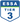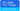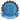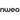# 4 Classroom Activities For Students To Learn Algebra [+ Downloadable Worksheets For Class]

All Posts

As students master primary mathematical concepts and advance to a new proficiency level, they are introduced to topics like algebra, geometry, and calculus in school. Diving into these new set of topics can be challenging for students as they require logical reasoning and problem-solving skills for the same.

Since algebraic concepts are introduced in class as early as 3rd grade, students require regular practise and extra efforts from teachers to be engaged in class. Therefore, to help them master algebraic concepts teachers can use math worksheets and introduce fun classroom activities to keep the students engaged and interested.

Check out these 4 classroom activities to tackle algebraic concepts and topics in an engaging way.

## 1. Riddle Me This‘Riddle Me This’ is a fun classroom activity for students. The game can be made easy or hard depending on the equations you select but essentially the motive is to solve the value of each algebraic equation and find the answer to the riddle. The student who manages to solve all the equations and find the answer to the riddle wins the game!

## 2. Hexagon Calculation GameThis hexagon puzzle is an algebra challenge that can be played by multiple players. It involves rolling a dice to work out the value of an algebraic expression. Students take turns rolling the dice and the number rolled becomes the value of ‘n’. The player then gets one chance to choose a hexagon and solve the equation using the assigned value of ‘n’. At the end of the game, the student with the most number of solved hexagons wins. This classroom activity also gives students a chance to work and have fun together in class.

Also read: 4 Fun Classroom Activities For Students To Learn Fractions.

## 3. Balance MathBalance Math is an innovative way to teach students how to solve simple linear equations. For this classroom activity, students need to find out the missing number to balance the pan. When both sides weigh the same, the pan stays balanced. Working on these pan balance problems is a great way to improve logical reasoning skills.

## 4. ProdigyTeachers also find Prodigy very helpful when it comes to teaching algebraic equations. Prodigy can be used as a regular classroom activity for students to solve fun puzzles and learn algebra faster. Since Prodigy is a free game-based math learning platform for students from grades 1-8, it is curriculum-aligned to present complex puzzles only when the student is ready to tackle the problems. So when it comes to algebra, your students won’t get anxious about solving difficult equations because they proceed to the next lesson only when they have mastered the current level.

Learning a new and challenging concept like algebra can be daunting for students, especially if they have math anxiety. Therefore, while introducing a new topic in class, it is important that teachers create an engaging environment that helps students to overcome difficulties, motivates them to actively participate in class, and improve their math skills.

Implement these 5 fun classroom activities in your class and make it a part of your class routine.••••